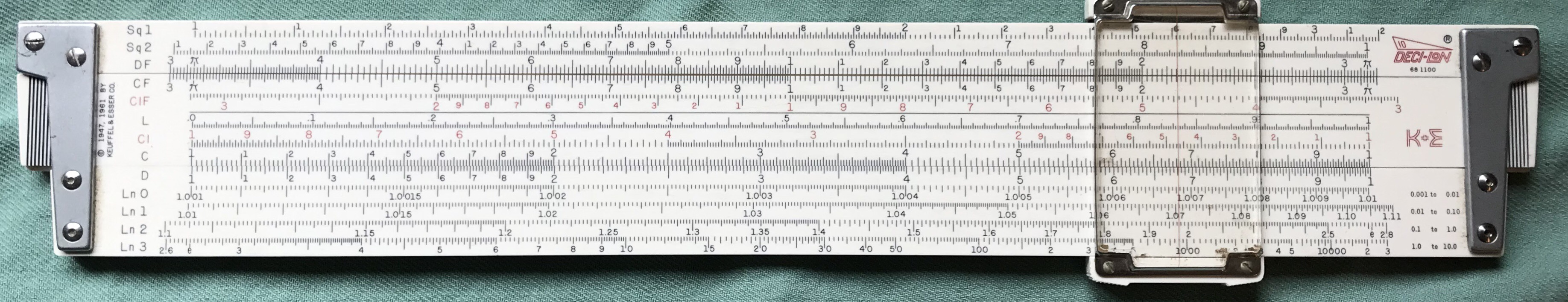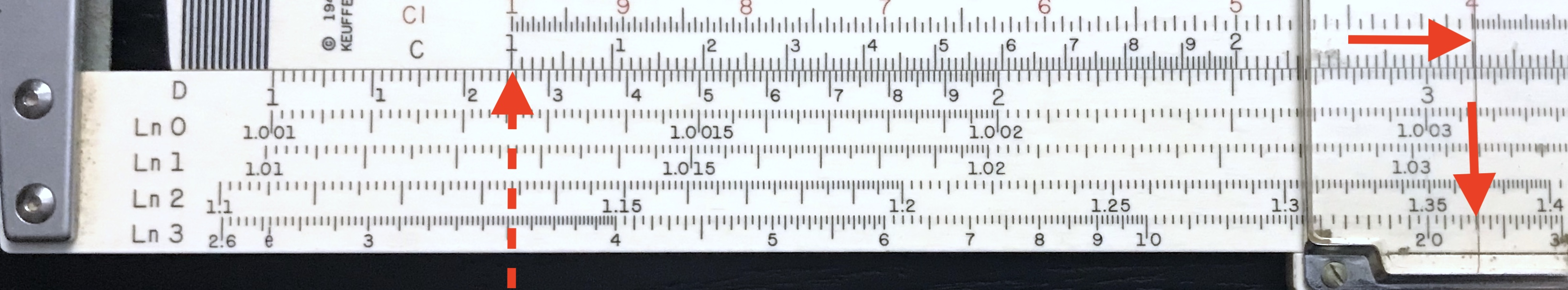## 3.8 Log-Log Scales

In the section entitled The Log Scale – L we discussed how the L scale can be used to find numbers raised to arbitrary powers. This involved looking up a log, multiplying the result by a number, then looking up what number has that log. Special scales called “log log” scales were created to make this a simpler operation. Suppose we seek a number $$z$$ which is given by a number $$x$$ taken to the power of $$y:$$ $z = x^y.$

If we take the logarithm of each side,

$\log z = \log x^y = y \times \log x,$ and by taking the logarithm again we find that

$\log \log z = \log y + \log\log x.$ Since we already have scales on the slide rule proportional to $$\log y$$ (the C scale, for instance), then by adding a scale that is proportional to the $$\log\log$$ of a number (that is, the logarithm of the logarithm of $$x$$) we can perform the exponential operation essentially in one step.

An example of a log-log scale is shown here (on the DeciLon slide rule, by K&E):Log-Log scales at the bottom of the DeciLon rule.

Suppose we want to compute $$3.5^{2.5}$$. Find the log-log scale (called Ln on this rule, it can also be designated by LL, or N, or perhaps other labels) at the bottom which has 3.5 in it. Note that these scales do not automatically repeat like standard “log” scales. So, you have to find the scale with the actual number of interest on it. On the rule we are using we find the number “3.5” on the Ln3 scale at the very bottom. Now, by placing the 1 on the C scale directly above 3.5 on the Ln3 scale, and sliding the cursor over to 2.5 on the C scale, we can read off “22.9” on the Ln3 scale directly. As you can readily imagine, we have added the log of 2.5 to the log-log of 3.5 to get the log-log of 22.9. Done!Exponentiation calculation using the Log-Log scale.

Well, we were actually a bit lucky there. First of all, perhaps 23 is as close a guess as one can read on this scale. The log-log scales aren’t always easy to read accurately, especially on the right-hand side. But also, one will often find that the answer appears to be off scale. Just as in multiplication and division problems, the ends of the slide can be exchanged when the result is off scale, after which the result can be read on the next log-log scale in the series. For each new log-log scale with larger values along it, the C/D scales represent an additional factor of 10 in the exponent. For example, had we wanted to take $$3.5^{0.25}$$, we would find the answer on the Ln2 scale, directly above our previous answer: approximately 1.368.

As another example, consider a calculation of the number $$1.065^{35}$$. With the cursor at 1.065 on the Ln1 (or LL1) scale, we must move the cursor to the left to reach 3.5; the result $$1.065^{3.5}$$ will now be found one scale higher, on the Ln2 (or LL2) scale: about 1.246. Our desired result $$1.065^{35}$$ is found on the Ln3 (or LL3) scale: approximately 9.06.

Of the 272 slide rules presently in the Collection, approximately 39% of them have log-log scales. More details about the creation and use of log-log scales can be found in the vignette Power Rules. Also, several very good synopses of the use of log-log scales to find numbers raised to powers can be found online; one Log-Log example page is based upon Pickett slide rules and covers the basics.

But a very important point that needs to be made in our present discussion is that the bases used in the log-log scales can be mixed. For instance, if $$z = x^y$$ and one takes the natural logarithm (Base $$e:~~$$ $$\ln x \equiv \log_e x$$ ), then $\ln z = y \times \ln x$ since the general rules of logarithms still apply. But then, taking the common (Base 10) log of the result, we find $\log \ln z = \log y + \log \ln x.$

Since the C scale utilizes Base 10, then the mix of logarithm bases still allows powers of numbers to be found using the exact same technique described above – this is because the number $$x$$ and the number $$z$$ are both read from the same scale or set of scales.

But now let’s let $$x= e$$ in our equation above. Then $\log \ln z = \log y + \log \ln e = \log y + 0 = \log y.$
In this case, $$\ln z = y$$, or $$z = e^y$$. So with these mixed bases, if a number $$y$$ is read on the D scale, then $$e^y$$ will be on the log-log scale. Hence such mixed-base log-log scales can be used to take numbers to arbitrary powers as well as to read values of the exponential function for a wide range of arguments. Additionally, by using the scales in reverse, finding the power that $$e$$ must be raised to in order to arrive at a particular number is equivalent to finding the natural logarithm of that number. The log-log scales can be used for all three of these tasks.

Look back at the figure above to view the Ln3 scale again. At the left end one can find the “eGauge Mark. It is located directly below the value of “1” on the D scale. This shows us that the Ln scales are mixed-base on this rule, since if the D scale has value $$x$$, with $$1\le x\le 10$$, then this Ln3 scale will correspond to $$e^x$$. The Ln2 scale will give $$e^{x/10}$$, Ln1 will be $$e^{x/100}$$, and Ln0 will be $$e^{x/1000}$$.

The earliest slide rules with log-log scales usually only had LL1 (or LL0) through LL3. If one needed numbers like, say, $$e^{-3}$$, then one would find $$e^3$$, record or remember that value, and then use the CI scale to find the inverse. Eventually scales of $$e^{-x}$$ were added, typically labeled LL03, LL02, LL01, and LL00 for direct reading of such values. They can also be used for the evaluation of hyperbolic trig functions.